# Excel COUNTIF Function – How to Use

As the name suggests Excel COUNTIF Function is a combination of Count and IF formula. In plain English, COUNTIF Function can be described as a formula that can be used for counting the number of cells that fulfill a particular condition, within a predefined range.

### How Excel Defines COUNTIF Function:

Microsoft Excel defines COUNTIF as a formula that, “Counts the number of cells within a range that meet the given condition”.

This definition clearly explains that: COUNTIF Function is a better and sophisticated type of COUNT formula that gives you the control over, which cells you wish to count.

### Syntax of Excel COUNTIF Formula:

Excel COUNTIF formula can be written as follows:

`=COUNTIF(range , criteria)`

Here ‘`range`’ specifies the range of cells over which you want to apply the ‘`criteria`‘.

`criteria`’ specifies the condition that a particular cell content should meet to be counted.

### How to Use COUNTIF in Excel:

Now, let’s see how to use COUNTIF function in Excel.

Let’s consider, we have an Employee table as shown in the below image.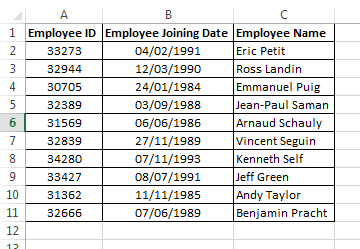Objective: From the above table, our objective is to find the number of employees who have joined before 1990.

So, we will try to use the COUNTIF Formula to find the result.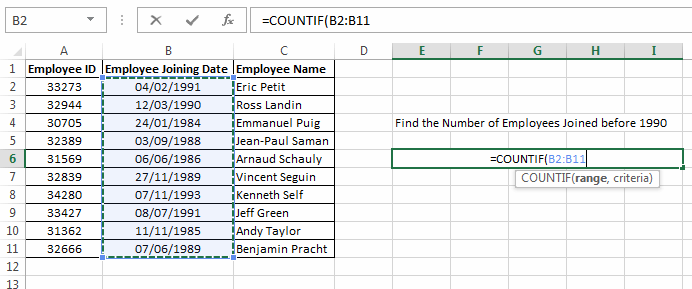range: In this case, ‘`range`’ will be “B2:B11”, as on these cells we have to apply the ‘`criteria`’.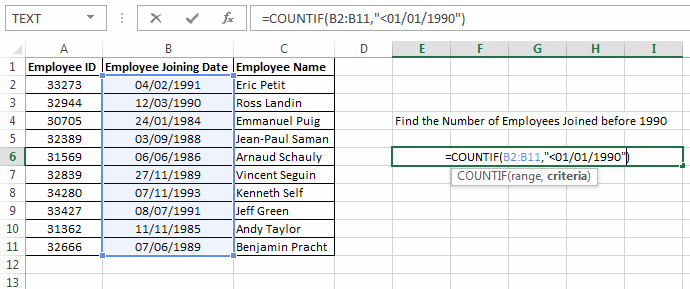criteria: In this case, ‘ `criteria`’ is “<01/01/1990”. This specifies that we want to count only those employees that are joined before 1st January 1990.This results into 6, which means there are 6 employees that have joined before 1990.

### Few important facts about COUNTIF formula:

1. COUNTIF formula only accepts a solid range, you cannot give multiple broken ranges to it. For example: COUNTIF cannot be written as `=COUNTIF(A1:A4 , A6:A8, ">0")`. You must use a single range like `=COUNTIF(A1:A8, ">0")`.

2. COUNTIF can accept wildcard characters (like “*” and “?”) in the ‘`criteria`’ argument. This means that you can write a COUNTIF as `=COUNTIF(D1:D15, "*o*")`. This will count all the cells containing “o” character, within the D1:D5 range.

3. As you know, the output of COUNTIF is an integer so you can also add two COUNTIF functions. For example: if you want to find the cells with value as “1” and cells with value as “2”, so you can use COUNTIF as `=COUNTIF(A1:A10,"1")+COUNTIF(A1:A10,"2")`.

4. COUNTIF throws a `#NAME?` error, if you supply an incorrect range to it .

### Few Basic Level Examples of COUNTIF Function: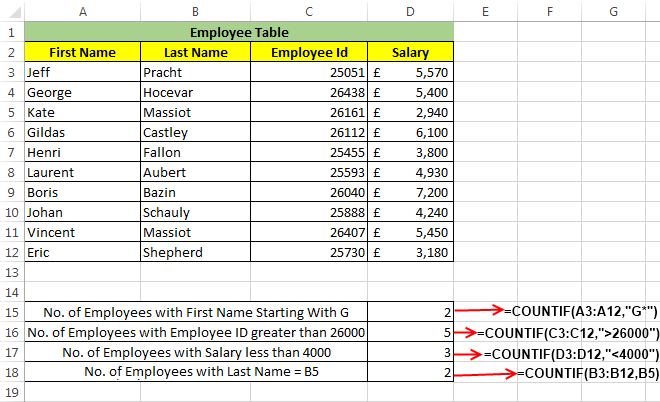In the above image, I have used an Employee table to depict how COUNTIF function can be used.

Example 1: In the first example, I have used Excel COUNTIF formula for finding the number of employees whose first name starts with “G”.

For this I have used a formula as `=COUNTIF(A3:A12,"G*")`.

Here, the COUNTIF Function scans the whole range from A3:A12 and tries to find a pattern “G*” (‘*’ is a wildcard operator which denotes any number of characters). The resultant is 2, as there are only two persons in the specified range whose first name starts with G.

Example 2: In the second example, I have used a COUNTIF function to find the cells which contain an Employee ID value greater than “26000”.

To accomplish this I have used a formula `=COUNTIF(C3:C12,">26000")`.

This formula searches the specified range for a value that specifies the criteria (i.e. >26000). So, the result is 5 as only 5 employees have an Employee ID greater than 26000.

Example 3: In the third example, I have fetched the number of employees whose salary is less than 4000.

To get this, I have again used a COUNTIF formula as `=COUNTIF(D3:D12,"<4000")`.

So, here the COUNTIF counts only those cells where salary range i.e. D3:D12 has a value less than 4000 and the resultant is 3.

Example 4: In the fourth example, I have used a formula `=COUNTIF(B3:B12,B5)` to find the number of cells equal to the value of the cell B5 (i.e. “Massiot”), in the range B3:B15.

Here, first the COUNTIF function finds the value at B5 cell and then it compares all the cells within the specified range with this value.

The resultant is 2 as only two records match the value at B5 cell.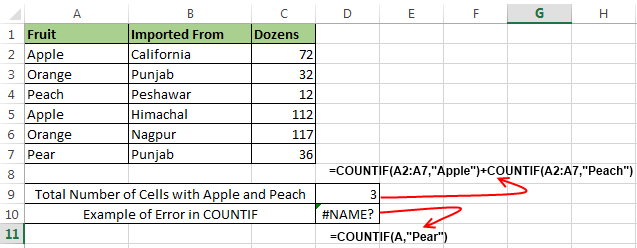Example 5: In the above example, I had to find the total count of cells that contain “Apple” or “Peach”.

This can be easily done by adding the resultants of two COUNTIF statements like: `=COUNTIF(A2:A7,"Apple")+COUNTIF(A2:A7,"Peach")`.

The first COUNTIF statement gives the number of cells with value equal to “Apple” and the second statement gives the count of cells with “Peach”. And hence the output comes out as 2+1=3.

Example 6: In this example (i.e. `=COUNTIF(A,"Pear")`), I have tried to show you what happens if you enter an incorrect range in COUNTIF function.

In such cases it throws a `#NAME?` error.

### Few Advanced Examples of COUNTIF Function:

Now, let’s see some practical examples of COUNTIF Function.

Example 7: Finding duplicate values using COUNTIF function.

Let’s say we have a table as below, and we have to find the duplicate records in it.For finding the duplicate records, we have used the formula: `=COUNTIF(\$A\$2:\$A\$16,A2)>1`

When this formula encounters a duplicate record it returns TRUE, while FALSE means that the record is Unique.If you are wondering what these dollar (\$) signs are doing in this formula, then you should read this post.

Recommended Reading: Find and Delete Duplicate cells in Excel

Example 8: Use COUNTIF formula for generating the sorting order of a list.

Let’s consider we have a list as below.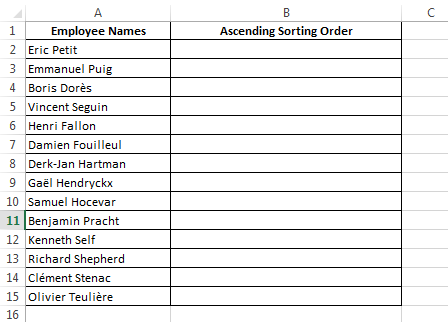Now, If we just want to know the alphabetic sorting order (in ascending order) of the employee names, then we can use the formula: `=COUNTIF(\$A\$2:\$A\$15,"<="&A2)`

See the below image, to see this formula in action.As you can see, that this formula simply generates a number in-front of every employee. This number is the sorting order (in ascending sort) of the Employee Names.

Recommended Reading: How to alphabetize a list in Excel

So, this was all from my side. Do share your ideas and experiences related to Excel COUNTIF Function in the below comments section.About Ankit Kaul

Ankit is the founder of Excel Trick. He is tech Geek who loves to sit in front of his square headed girlfriend (his PC) all day long. :D. Ankit has a strong passion for learning Microsoft Excel. His only aim is to turn you guys into 'Excel Geeks'.